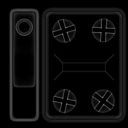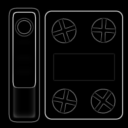# Normal Curvature node

Left Toolbar >Normal Nodes >Normal Curvature

The Normal Curvature node finds the average curvature between the normals around each pixel.

inputImg

outputCurvatureConvexConcave

## Procedure

### To generate the Curvature image from a Normal image:

1. On the left toolbar choose Normal Nodes > Normal Curvature.>2. Drag the Normal Curvature icon into the Node Graph.3. Connect a Normal image to the input socket in the Normal Curvature node.

4. Connect the Output: Curvature, Convex or Concave.

A Normal Image.

## Outputs

Curvature
A monochromatic image where 50% gray represents flat areas, white represents convex areas, and black represents concave areas.

Convex A monochromatic image where black represents flat areas and white represents convex areas.

Concave A monochromatic image where black represents flat areas and white represents concave areas.

## Attributes PanelRadius Defines a circular area around each pixel that is measured for curvature.